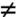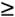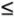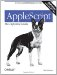# Section 15.4. Comparison Operators

### 15.4. Comparison Operators

The comparison operators test whether one operand is the same as the other, or whether, if they can be ordered, they are ordered in a given direction. The result is a boolean.

The nature of comparisons involving strings can be influenced by a considering clause; see Chapter 19.

Lists are internally ordered, but records are not:

` {1, 2} = {2, 1} -- false {name:"Matt", age:"51"} = {age:"51", name:"Matt"} -- true `

The equality (=) and inequality () operators do false

In that example, the first operand is a list, the second operand is a number, and no coercion takes place. So the operands are not equal, and the comparison is false.

With the other comparison operators , operands must ultimately be a string, a number, or a date. The first operator is coerced to a string, a number, or a date, and then the second operator is coerced to match the datatype of the first:

` {"2"}2 -- In that example, the first operand is a list of one string, so it is coerced to a string. Now the second operand is coerced to a string; the two strings are equal and the comparison is true. Thus, although you cannot use the equality   operator to learn whether two values would be equal if implicitly coerced to the same datatype, you can work around the problem like this:  {"2"}2 and {"2}2 -- As noted in "Assignment and Retrieval" in Chapter 7, the equality operator is not overloaded as an assignment operator. This code will compile and run, but it won't assign 4 to x (it will report whether they are equal):  x = 4  = (is)equality equality Syntax  operand1 = operand2  Description Yields true if the operands are equal. No coercion is performed; different datatypes are not equal. Synonym is equal to has abbreviations equal, equals, and equal to. inequality inequality Syntax  operand1operand2  Description Yields true if the the operands are unequal. No coercion is performed; different datatypes are not equal. The not-equals sign is typed using Option-=. is not has abbreviation isn't. Synonym is not equal to has abbreviations is not equal, isn't equal, does not equal, and doesn't equal. There are no synonyms <> or !=. <less than less than Syntax  operand1 < operand2  Description Yields true if the first operand is less than the first. Synonyms are is less than (abbreviation less than) and comes before. >greater than greater than Syntax  operand1 > operand2  Description Yields TRue if the first operand is greater than the first. Synonyms are is greater than (abbreviation greater than) and comes after. less than or equal to less than or equal to Syntax  operand1operand2  Description Yields TRue if the first operand is less than or equal to the first. Abbreviation is <=, or thesymbol may be typed using Option-comma. Synonym is less than or equal to has abbreviations omitting is, to, or both. There are also synonyms does not come after and is not greater than. greater than or equal to greater than or equal to Syntax  operand1operand2  Description Yields true if the first operand is greater than or equal to the second. Abbreviation is >=, or thesymbol may be typed using Option-period. Synonym is greater than or equal to has abbreviations omitting is, to, or both. There are also synonyms does not come before and is not less than. `AppleScript: The Definitive Guide, 2nd Edition
ISBN: 0596102119
EAN: 2147483647
Year: 2006
Pages: 267
Authors: Matt Neuburg

Similar book on Amazon

flylib.com © 2008-2017.
If you may any questions please contact us: flylib@qtcs.net